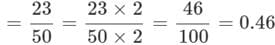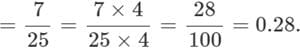Test: Decimals- 1

# Test: Decimals- 1

Test Description

## 10 Questions MCQ Test Mathematics for Class 5 | Test: Decimals- 1

Test: Decimals- 1 for Class 5 2023 is part of Mathematics for Class 5 preparation. The Test: Decimals- 1 questions and answers have been prepared according to the Class 5 exam syllabus.The Test: Decimals- 1 MCQs are made for Class 5 2023 Exam. Find important definitions, questions, notes, meanings, examples, exercises, MCQs and online tests for Test: Decimals- 1 below.
Solutions of Test: Decimals- 1 questions in English are available as part of our Mathematics for Class 5 for Class 5 & Test: Decimals- 1 solutions in Hindi for Mathematics for Class 5 course. Download more important topics, notes, lectures and mock test series for Class 5 Exam by signing up for free. Attempt Test: Decimals- 1 | 10 questions in 15 minutes | Mock test for Class 5 preparation | Free important questions MCQ to study Mathematics for Class 5 for Class 5 Exam | Download free PDF with solutions
 1 Crore+ students have signed up on EduRev. Have you?
Test: Decimals- 1 - Question 1

### Find the decimal number for "one and four tenths".

Detailed Solution for Test: Decimals- 1 - Question 1

1.4 one unit and four tenths = 1 + 4 / 10
= 1 + 0.4 = 1.4.

Test: Decimals- 1 - Question 2

### Find the decimal number for "Sixty Seven and Twenty Three One-Hundredths".

Detailed Solution for Test: Decimals- 1 - Question 2

Sixty Seven and Twenty Three One-Hundredths = 67 + 23 / 100
= 67 + 0.23 = 67.23.

Test: Decimals- 1 - Question 3

### Find the decimal number for "Twenty Three Fiftieths".

Detailed Solution for Test: Decimals- 1 - Question 3

Twenty Three FiftiethsTest: Decimals- 1 - Question 4

Find the decimal number for "Seven Twenty Fifths".

Detailed Solution for Test: Decimals- 1 - Question 4

Seven Twenty FifthTest: Decimals- 1 - Question 5

Find the decimal number for "12.78" rounded to the nearest tenth.

Detailed Solution for Test: Decimals- 1 - Question 5

12.78 rounded to nearest tenth = 12.8.
Since the digit on hundredth place 8 > 5, the tenth place is rounded by adding 1 to 7.

Test: Decimals- 1 - Question 6

Find the decimal number for "3.8439" rounded to the nearest hundredth.

Detailed Solution for Test: Decimals- 1 - Question 6

3.8439 rounded to nearest hundredth = 3.84.
Since the digit on thousandth place 3 < 5, the hundredth place remains unchanged.

Test: Decimals- 1 - Question 7

Round 12.6257 to three decimal places.

Detailed Solution for Test: Decimals- 1 - Question 7

12.6257 to three decimal places = 12.626.
Since the digit after 5 is 7 and 7 > 5, the thousandth place is increased by 1.

Test: Decimals- 1 - Question 8

Round 106.5 to the nearest whole number.

Detailed Solution for Test: Decimals- 1 - Question 8

106.5 rounded to nearest whole number = 107.
Since the digit after decimal is 5, the unit place is rounded by adding 1 to 6.

Test: Decimals- 1 - Question 9

Find the round 239.456 to the nearest hundred.

Detailed Solution for Test: Decimals- 1 - Question 9

"2" needs to be retained in the hundreds position.
The next digit is "3" which is less than 5, so no change is needed to "2".
∴ Required answer = 2 hundreds.

Test: Decimals- 1 - Question 10

Diana sells 12 garlands for Rs. 12.12. What is the cost of each garland?

Detailed Solution for Test: Decimals- 1 - Question 10

12.12 ÷ 12 = 1.01
∴ Cost of each garland = 1.01.

## Mathematics for Class 5

12 videos|55 docs|35 tests
 Use Code STAYHOME200 and get INR 200 additional OFF Use Coupon Code
Information about Test: Decimals- 1 Page
In this test you can find the Exam questions for Test: Decimals- 1 solved & explained in the simplest way possible. Besides giving Questions and answers for Test: Decimals- 1, EduRev gives you an ample number of Online tests for practice

## Mathematics for Class 5

12 videos|55 docs|35 tests